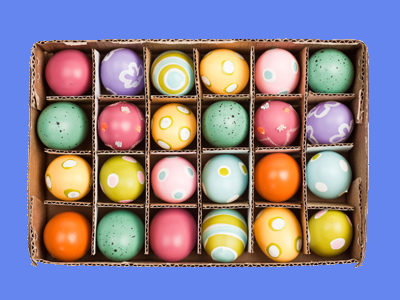6 x 4 = 24 and 4 x 6 = 24 as well!

# Multiply in Any Order

This Math quiz is called 'Multiply in Any Order' and it has been written by teachers to help you if you are studying the subject at elementary school. Playing educational quizzes is an enjoyable way to learn if you are in the 3rd, 4th or 5th grade - aged 8 to 11.

It costs only \$12.50 per month to play this quiz and over 3,500 others that help you with your school work. You can subscribe on the page at Join Us

When we multiply two numbers together, the two numbers being multiplied can be done in either order, and still give the same answer. This is known as 'cumulative property'. For example, 5 x 4 gives the same result as 4 x 5. This knowledge can be used to answer any questions involving times tables children are unfamiliar with (if they don't know 5 x 7, they may know 7 x 5 for instance). Cumulative property also applies when 3, 4 or any other group of numbers are to be multiplied. The calculation can be done in any order and the answer will be the same.

Try this quiz to see how much you know about multiplying in any order.

1.
What is 50 x 2?
52
100
25
70
2 x 50 is much easier to work out!
2.
5 x 6 gives the same answer as...
40 x 5
4 x 50
5 + 4
6 x 5
They both give an answer of 30
3.
Which of the following pairs give an answer of 60?
60 x 2 and 2 x 60
30 x 2 and 2 x 30
15 x 3 and 3 x 15
40 x 4 and 4 x 40
2 x 30 gives an answer of 60, as does 30 x 2
4.
Which number is missing?
? x 8 = 16
4
24
12
2
If you know that 8 x 2 = 16, then 2 x 8 = 16 also
5.
Which of the following pairs give an answer of 20?
20 x 2 and 2 x 20
4 x 5 and 5 x 4
10 x 4 and 4 x 10
10 x 20 and 20 x 10
4 x 5 = 20, as does 5 x 4
6.
Which number is missing?
20 x ? = 80
60
40
24
4
4 x 20 = 80 and 20 x 4 = 80 too
7.
What is 100 x 4?
410
40
104
400
Changing the order of the numbers makes this 4 x 100, which is easier to work out
8.
When you multiply two numbers...
you can multiply them in any order
you will get an answer of more than 50
you will also find the difference
When multiplying two numbers, it doesn't matter which way round they go, you will still get the same answer
9.
I don't know my 7 times table yet. How could I work out 5 x 7?
By quickly learning the 7 times table
By doing 7 x 5 instead
By adding 7 and 5 together
5 x 7 = 35 and 7 x 5 is 35 too
10.
What could make this calculation easier to work out?
5 x 12
Quickly learning the 12 times table